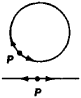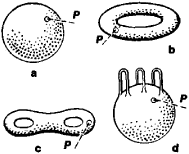# manifold

(redirected from manifoldly)
Also found in: Dictionary, Thesaurus.

## manifold

Formal
1. a chamber or pipe with a number of inlets or outlets used to collect or distribute a fluid. In an internal-combustion engine the inlet manifold carries the vaporized fuel from the carburettor to the inlet ports and the exhaust manifold carries the exhaust gases away
2. Maths
a. a collection of objects or a set
b. a topological space having specific properties
3. (in the philosophy of Kant) the totality of the separate elements of sensation which are then organized by the active mind and conceptualized as a perception of an external object
Collins Discovery Encyclopedia, 1st edition © HarperCollins Publishers 2005

## Manifold

Component that distributes the water in a home-run plumbing system. It has one inlet and many outlets, each of which feeds one fixture or appliance.
Illustrated Dictionary of Architecture Copyright © 2012, 2002, 1998 by The McGraw-Hill Companies, Inc. All rights reserved
The following article is from The Great Soviet Encyclopedia (1979). It might be outdated or ideologically biased.

## Manifold

a system of devices and equipment for the startup and continuous trouble-free operation of oil and gas wells. It consists of piping and connections, gates, valves, tee joints, fourway pieces, standpipes (risers), shock absorbers, small cocks, compensators, and bypasses. In flowing-well and compressor-lift oil production, the manifold is connected to the Christmas tree mainly by means of flange couplings and ends with the line of pipes supplying the output to the gauging devices.

## Manifold

a mathematical concept that refines and generalizes to any number of dimensions the concept of a curve or surface without singular points (that is, curves without points of self-intersection, end points, and the like and surfaces without self-intersections, boundaries, and so forth).

Examples of one-dimensional manifolds are a line, a parabola, a circle, an ellipse, and, in general, any curve at each point of which there exists a neighborhood that is a one-to-one bicontinuous (or, to use topological terminology, homeomorphic) image of an interval (the interior of a line segment). An interval is itself a one-dimensional manifold, although a line segment is not a manifold, since its end points lack such neighborhoods.

Two-dimensional manifolds include any region in the plane (for example, the interior of the circle x2 + y2 < r2), the plane itself, a paraboloid, a sphere, an ellipsoid, a torus, and others. Each of their points has a neighborhood homeomorphic to the interior of a circle. This requirement eliminates, for example, a conical surface (its vertex, which is common to its two nappes, lacks the type of neighborhood required). However, we distinguish a particular class of objects that do not satisfy this requirement—the manifolds with a boundary (for example, the closed circle x2 + y2r2).

Three-dimensional manifolds include ordinary Euclidean space as well as any open set in Euclidean space. A characteristic feature of three-dimensional manifolds is that each point of such a manifold has a neighborhood homeomorphic to the interior of a sphere.Figure 1. One-dimensional manifolds

Manifolds are either closed or open (see below for definition). In the case of one dimension, every closed manifold is homeomorphic to a circle, and every open manifold, to a line (Figure 1 depicts one-dimensional manifolds and neighborhoods of a point P in each of them). The closed manifolds in the case of two dimensions are already quite varied. They belong to infinitely many topological types: the sphere, which is a surface of genus 0 (Figure 2,a); the torus, which is a surface of genus 1 (Figure 2,b); the two-holed doughnut, which is a surface of genus 2 (Figure 2,c); and, in general, the “sphere with n handles,” which is a surface of genus n (Figure 2,d depicts such a surface for n = 3). All topological types of closed two-dimensional orientable manifolds are exhausted by these examples. There exists an infinite number of closed two-dimensional nonorientable manifolds, namely, the one-sided surfaces, for example, the projective plane and the one-sided torus (Klein bottle).

We also have a classification of open two-dimensional mani-folds. At this time (1974), we have no complete classification of three-dimensional manifolds, or even of closed three-dimensional manifolds.Figure 2. Examples of closed two-dimensional manifolds

A manifold of n-dimensions (or n-dimensional manifold) is a Hausdorff topological space having the following properties: (1) each point in it has a neighborhood homeomorphic to the interior of an n-dimensional sphere and (2) the entire space can be represented as a sum of a finite or countably infinite set of such neighborhoods. A manifold is said to be closed if it is compact and open if it is not. The requirement that a manifold be connected, that is, that every two points in it can be connected by a continuous arc, is sometimes added to the definition of a manifold.

The concept of a manifold of any (natural) number of dimensions n was introduced into mathematics to meet the highly varied needs of geometry, mathematical analysis, mechanics, and physics. The importance of a sufficiently broad interpretation of a manifold as a topological space is that any kind of object, for example, line, sphere, and matrix, can be points of manifolds thus defined.

A special kind of manifold is a smooth, or differentiable, mani-fold. It is possible to study differentiable functions on a smooth manifold, as well as differentiable mappings of a smooth mani-fold into itself or into other smooth manifolds. Smooth mani-folds are of particular importance in modern mathematics since they are the most widely used in applications and in related fields (for example, configuration spaces and phase spaces in mechanics and physics). It is possible to introduce a metric on a smooth manifold, thus converting it into a Riemannian manifold. On such manifolds it is possible to introduce a differential geometry. For example, by introducing a metric in the configuration space of a mechanical system, it is possible to interpret trajectories of motions as geodetic lines in this space. A manifold for whose elements there is defined a (differentiable) multiplication that makes the manifold into a group is said to be a Lie group.

The concept of manifold plays a major role in the theory of algebraic functions, continuous groups, and other fields. The properties of a manifold that remain invariant under topological transformations (topological properties) are essential in these applications. They include the orientability or nonorientability of a manifold. The study of these properties constitutes one of the most important problems in topology.

### REFERENCES

Aleksandrov, P. S., and V. A. Efremovich. Ocherk osnovnykh poniatii topologii. Moscow-Leningrad, 1936.
Aleksandrov, P. S. Kombinatornaia topologiia. Moscow-Leningrad, 1947.
Lang, S. Vvedenie v teoriiu differentiruemykh mnogoobrazii. Moscow, 1967. (Translated from English).

N. V. EFIMOV

The Great Soviet Encyclopedia, 3rd Edition (1970-1979). © 2010 The Gale Group, Inc. All rights reserved.

## manifold

[′man·ə‚fōld]
(engineering)
The branch pipe arrangement which connects the valve parts of a multicylinder engine to a single carburetor or to a muffler.
(mathematics)
A topological space which is locally euclidean; there are four types: topological, piecewise linear, differentiable, and complex, depending on whether the local coordinate systems are obtained from continuous, piecewise linear, differentiable, or complex analytic functions of those in euclidean space; intuitively, a surface.
McGraw-Hill Dictionary of Scientific & Technical Terms, 6E, Copyright © 2003 by The McGraw-Hill Companies, Inc.

## manifold

A section of duct, a fitting, or a pipe with a number of branches which are close together.
McGraw-Hill Dictionary of Architecture and Construction. Copyright © 2003 by McGraw-Hill Companies, Inc.

## manifold

A chamber having a number of outlets for distributing fluids or gases. Examples: fuel manifold, exhaust manifold, hydraulic manifold, inlet manifold, engine manifold, etc. See exhaust manifold and oxygen manifold.
An Illustrated Dictionary of Aviation Copyright © 2005 by The McGraw-Hill Companies, Inc. All rights reserved
Site: Follow: Share:
Open / Close